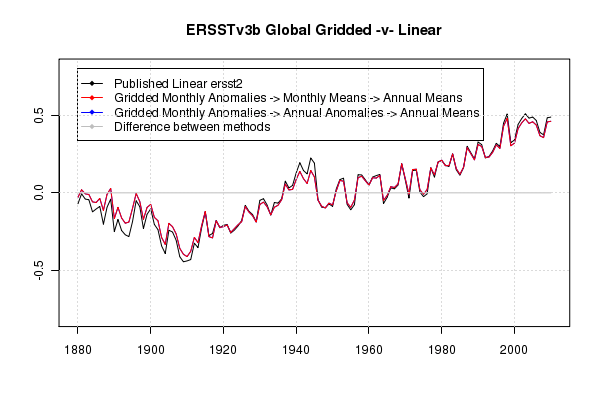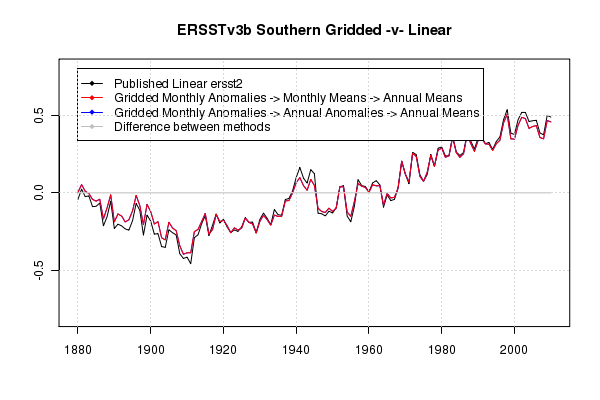2011 March 1Here we use the same frequency analysis tools used for HadSST2 to look at ERSSTv3b.

 Fourier Analysis annual monthly Global 160, 80, 53.3 160, 80, 53.3 Northern Hemisphere 160, 80, 53.3, 32 160, 80, 53.3, 32 Southern Hemisphere 160, 53 160, 53

I tweaked the function that produced the global mean. It now uses a “weighted cell” method rather than a “weighted latitude.” There are reasons I prefer the latter for global or hemispheric means, but this provides a very close match to the NCDC linear trend and will be more suitable for regional work. Using the area weighted rather than latitude weighted method, there is no significant difference between annual and monthly series in regards to the Fourier intensity tests.

`getRasterLatMean <- function(r) {`

``` rows = dim(r) cols = dim(r) wcell <- area(r,na.rm=F) # cells wlat <- extract(wcell,seq(1,((rows-1)*cols+1),cols)) # lat bands ```

``` m <- mask(wcell,r) r1 <- r*wcell r2 <- extract(r1,ncell(r1)) return(sum(extract(r1,1:ncell(r1)),na.rm=T)/sum(extract(m,1:ncell(m)),na.rm=T)) }```